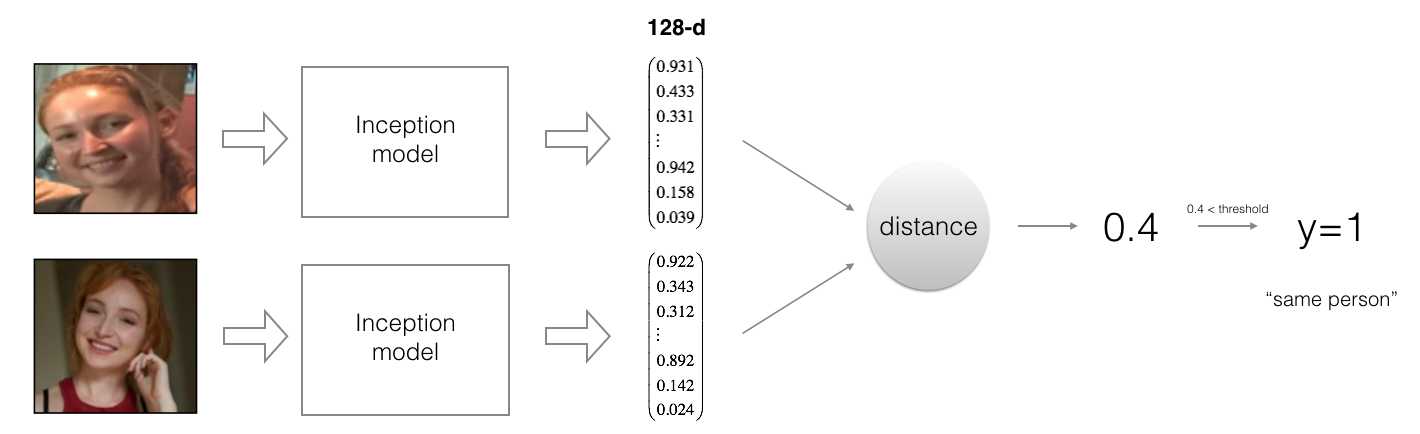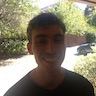# AI 学习之 FaceNet 人脸识别

### 环境安装

• 1，打开Anaconda prompt
• 2，执行activate tensorflow命令
• 3，执行pip install keras==2.0.7命令
• 4，执行pip install opencv-python命令

Ubuntu操作系统，安装opencv-python命令

# 如果 pip  install opencv-python 报错，请用pip3安装
pip3 install opencv-python

 apt install -y libsm6 libxext6

apt-get install libxrender1

## 人脸验证 vs 人脸识别

• 人脸验证 - 例如，我们在火车站可以让机器扫描身份证并用脸对着摄像头后，就可以通过闸机了。因为系统验证了摄像头面前的人和身份证是同一个人。人脸验证是“一对一”的验证，例如验证摄像头面前的人是否是身份证上的人，又例如验证手机摄像头面前的人是否与之前添加面部解锁时留存的照片是同一个人。

• 人脸识别 - 人脸识别就是即使没有提供身份证，系统也能识别出摄像头面前这个人是谁。

from keras.models import Sequential
from keras.layers import Conv2D, ZeroPadding2D, Activation, Input, concatenate
from keras.models import Model
from keras.layers.normalization import BatchNormalization
from keras.layers.pooling import MaxPooling2D, AveragePooling2D
from keras.layers.merge import Concatenate
from keras.layers.core import Lambda, Flatten, Dense
from keras.initializers import glorot_uniform
from keras.engine.topology import Layer
from keras import backend as K
K.set_image_data_format('channels_first')
import cv2
import os
import numpy as np
from numpy import genfromtxt
#import pandas as pd
import tensorflow as tf
from fr_utils import *
from inception_blocks_v2 import *

%matplotlib inline

#np.set_printoptions(threshold=np.nan)
Using TensorFlow backend.
/usr/local/lib/python3.6/dist-packages/tensorflow/python/framework/dtypes.py:458: FutureWarning: Passing (type, 1) or '1type' as a synonym of type is deprecated; in a future version of numpy, it will be understood as (type, (1,)) / '(1,)type'.
_np_qint8 = np.dtype([("qint8", np.int8, 1)])
/usr/local/lib/python3.6/dist-packages/tensorflow/python/framework/dtypes.py:459: FutureWarning: Passing (type, 1) or '1type' as a synonym of type is deprecated; in a future version of numpy, it will be understood as (type, (1,)) / '(1,)type'.
_np_quint8 = np.dtype([("quint8", np.uint8, 1)])
/usr/local/lib/python3.6/dist-packages/tensorflow/python/framework/dtypes.py:460: FutureWarning: Passing (type, 1) or '1type' as a synonym of type is deprecated; in a future version of numpy, it will be understood as (type, (1,)) / '(1,)type'.
_np_qint16 = np.dtype([("qint16", np.int16, 1)])
/usr/local/lib/python3.6/dist-packages/tensorflow/python/framework/dtypes.py:461: FutureWarning: Passing (type, 1) or '1type' as a synonym of type is deprecated; in a future version of numpy, it will be understood as (type, (1,)) / '(1,)type'.
_np_quint16 = np.dtype([("quint16", np.uint16, 1)])
/usr/local/lib/python3.6/dist-packages/tensorflow/python/framework/dtypes.py:462: FutureWarning: Passing (type, 1) or '1type' as a synonym of type is deprecated; in a future version of numpy, it will be understood as (type, (1,)) / '(1,)type'.
_np_qint32 = np.dtype([("qint32", np.int32, 1)])
/usr/local/lib/python3.6/dist-packages/tensorflow/python/framework/dtypes.py:465: FutureWarning: Passing (type, 1) or '1type' as a synonym of type is deprecated; in a future version of numpy, it will be understood as (type, (1,)) / '(1,)type'.
np_resource = np.dtype([("resource", np.ubyte, 1)])

## 1 - 将图片编码成128维的向量

### 1.1 - 使用卷积网络来进行编码

• 这个网络要求输入图片的像素是96x96。所以输入是 $(m, n_C, n_H, n_W) = (m, 3, 96, 96)$ 。
• 它的输出的维度是$(m, 128)$。

FRmodel = faceRecoModel(input_shape=(3, 96, 96))
print("Total Params:", FRmodel.count_params())
Total Params: 3743280

FaceNet最后一层是一个全连接层，这一层中有128个神经元，所以最后会输入128个激活值，这个128维的向量就代表了输入的图片，也就是将一张图片编码（encoding）成了128维的向量。然后我们就可以使用这个编码后的向量来对比两张脸部图片:• 如果两张图片是同一个人，这两张图片的编码非常接近，那么编码是优秀的
• 如果两张图片是不同的人，这两张图片的编码相差很远，那么编码是优秀的

### 1.2 - 三元组损失• A 是指"Anchor"图像——一个人的图像
• P 是指"Positive"图像——这张图像里面的人与A是同一个人
• N 是指"Negative"图像——这张图像里面的人与A不是同一个人

$$\mid \mid f(A^{(i)}) - f(P^{(i)}) \mid \mid_2^2 + \alpha < \mid \mid f(A^{(i)}) - f(N^{(i)}) \mid \mid_2^2$$$$\mathcal{J} = \sum^{N}_{i=1} \large[ \small \underbrace{\mid \mid f(A^{(i)}) - f(P^{(i)}) \mid \mid_2^2}_\text{(1)} - \underbrace{\mid \mid f(A^{(i)}) - f(N^{(i)}) \mid \mid_2^2}_\text{(2)} + \alpha \large ] \small_+ \tag{3}$$

• 上面公式中标了(1)的那一块表示A与P的编码差异。这个值越小越好。
• 上面公式中标了(2)的那一块表示A与N的编码差异。这个值越大越好。
• $\alpha$是一个超参数，是需要我们自己慢慢调参的。在本文中我们使用$\alpha = 0.2$。
# 实现三元组损失函数

def triplet_loss(y_true, y_pred, alpha = 0.2):
"""
参数:
y_true -- 这个参数我们暂时不用
y_pred -- 这是一个python列表，里面包含了3个对象,分别是A,P,N的编码。

返回值:
loss -- 损失值
"""

anchor, positive, negative = y_pred, y_pred, y_pred

# 计算A与P的编码差异，就是公式中标（1）的那块
pos_dist = tf.reduce_sum(tf.square(tf.subtract(anchor, positive)))
# 计算A与N的编码差异，就是公式中标（2）的那块
neg_dist = tf.reduce_sum(tf.square(tf.subtract(anchor, negative)))
# 根据两组编码差异来计算损失
loss = tf.maximum(tf.reduce_mean(basic_loss), 0.0)

return loss
with tf.Session() as test:
tf.set_random_seed(1)
y_true = (None, None, None)
y_pred = (tf.random_normal([3, 128], mean=6, stddev=0.1, seed = 1),
tf.random_normal([3, 128], mean=1, stddev=1, seed = 1),
tf.random_normal([3, 128], mean=3, stddev=4, seed = 1))
loss = triplet_loss(y_true, y_pred)

print("loss = " + str(loss.eval()))
loss = 350.02618

## 2 - 加载已经训练好了的模型

FRmodel.compile(optimizer = 'adam', loss = triplet_loss, metrics = ['accuracy'])
load_weights_from_FaceNet(FRmodel)

## 3 - 应用训练好的FaceNet模型

### 3.1 - 人脸验证

database = {}

# 图片被转为 128维度的向量
database["danielle"] = img_to_encoding("images/danielle.png", FRmodel)
print(database["danielle"])

database["younes"] = img_to_encoding("images/younes.jpg", FRmodel)
database["tian"] = img_to_encoding("images/tian.jpg", FRmodel)
database["andrew"] = img_to_encoding("images/andrew.jpg", FRmodel)
database["kian"] = img_to_encoding("images/kian.jpg", FRmodel)
database["dan"] = img_to_encoding("images/dan.jpg", FRmodel)
database["sebastiano"] = img_to_encoding("images/sebastiano.jpg", FRmodel)
database["bertrand"] = img_to_encoding("images/bertrand.jpg", FRmodel)
database["kevin"] = img_to_encoding("images/kevin.jpg", FRmodel)
database["felix"] = img_to_encoding("images/felix.jpg", FRmodel)
database["benoit"] = img_to_encoding("images/benoit.jpg", FRmodel)
database["arnaud"] = img_to_encoding("images/arnaud.jpg", FRmodel)
[[ 0.11276133  0.03207601  0.13590078  0.11822379  0.07636504  0.13431978
0.14880915 -0.13990873 -0.01258973 -0.06264802 -0.06895615  0.05656987
-0.00625059  0.00522547  0.07190287 -0.05568053  0.04310033  0.04391421
-0.04496383 -0.0464243  -0.00843207  0.12305687 -0.0955635   0.17989582
0.01794844 -0.10260746 -0.14342976 -0.03599926 -0.04165843  0.13982902
-0.04188614  0.11941336 -0.12171154  0.05106764  0.08596217 -0.05465613
0.08568984 -0.06256222  0.00192188 -0.03967048  0.1094245   0.00864273
0.06470723 -0.19549362 -0.03102044  0.05460501  0.00480903 -0.02807491
-0.02172411 -0.03455152  0.150454   -0.07591686  0.16888484  0.01827822
0.10440756  0.02885913 -0.00706925  0.07838197  0.01713117  0.01476892
0.01493397  0.11768679 -0.09003265 -0.18028447  0.06014766  0.08900282
0.06730765 -0.0721844  -0.17830266 -0.01774505  0.01251065  0.04428384
0.00113512 -0.01007593  0.00903066  0.14183906  0.02696196 -0.09450618
0.05239265  0.00276199  0.10742998  0.10096071  0.02834628 -0.02422235
0.09584816 -0.04698139  0.00972352 -0.05569498 -0.18729015  0.13399333
0.0195455  -0.04999194  0.08253612  0.05580702 -0.17049019  0.06091601
0.05819915 -0.06772915  0.14484575  0.06185556  0.1364086   0.06831186
-0.01017165  0.06564528 -0.10523929 -0.04865944  0.00053396 -0.06757927
-0.1512183   0.10154685  0.08557729 -0.01880001  0.04089973 -0.09515373
-0.04399641  0.03859749 -0.17101029  0.10744184 -0.19870925 -0.00651667
0.02781366 -0.02077368 -0.01132978 -0.08182196  0.06889162  0.16284062
-0.05861037 -0.14208524]]

# 验证人脸

def verify(image_path, identity, database, model):
"""

参数:
image_path -- 需要被验证的人脸图片地址，也就是摄像头拍摄到的人脸图片
identity -- 人名，也就是扫描ID后得到的人名
database -- 有权开门的人脸数据库
model -- 前面加载的已经训练好了的FaceNet模型

返回值:
dist -- 返回image_path的人脸编码与identity指定的人名的人脸编码的差异
door_open -- 如果返回True, 就表示image_path和identity是同一个人，那么验证通过，门自动为他开启
"""

encoding = img_to_encoding(image_path, model)

# 对比identity关联的图片编码与image_path关联的图片编码的差异
dist = np.linalg.norm(encoding-database[identity])

# 差异小于0.7，就表明是同一个人。
# 当然，因为我们的程序的目的是教学，所以精度不高，实际应用中会取比0.7更小的阈值
if dist < 0.7:
print("It's " + str(identity) + ", welcome home!")
door_open = True
else:
print("It's not " + str(identity) + ", please go away")
door_open = False

return dist, door_openverify("images/camera_0.jpg", "younes", database, FRmodel)
It's younes, welcome home!

(0.67100745, True)verify("images/camera_2.jpg", "kian", database, FRmodel)
It's not kian, please go away

(0.85800153, False)

### 3.2 - 人脸识别

# 人脸识别

def who_is_it(image_path, database, model):
"""
参数:
image_path -- 需要被识别的人脸图像的地址
database -- 有权开门的人脸数据库
model -- 前面加载的已经训练好了的FaceNet模型

Returns:
min_dist -- 最小差异。我们会一个个与数据库中的人脸编码进行对比，找到与待识别的图像差异最小的那个图像
identity -- 差异最小的人脸图像关联的那个人的名字
"""

encoding = img_to_encoding(image_path, model)

min_dist = 100

# 与数据库中的人脸一个个的进行对比
for (name, db_enc) in database.items():

# 进行对比
dist = np.linalg.norm(encoding-db_enc)

# 保存差异最小的那个
if dist < min_dist:
min_dist = dist
identity = name

if min_dist > 0.7:
print("Not in the database.")
else:
print ("it's " + str(identity) + ", the distance is " + str(min_dist))

return min_dist, identity

who_is_it("images/camera_4.jpg", database, FRmodel)
it's dan, the distance is 0.2523855

(0.2523855, 'dan')

• 在数据库中保存一个人的多张照片（同一个人不同光照环境下的多张图片，不同时间段的多张图片等等）。也就是说，拿输入图片与数据库中同一个人多张图像进行验证对比，这样可以提升精准度。
• 将图片中的人脸部分截取出来。也就是说，只对比人脸部分。这样就减少了其它部分，例如衣服，背景等等像素的干扰。这样可以大大的提升精准度。你在生活中应该也看到了，当我们对准人脸识别摄像头时，屏幕上我们人脸的部分总有一个方框跟随着，它就是在定位并截取我们的人脸部分图像。